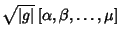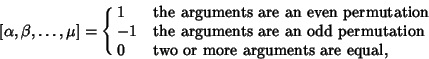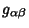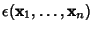## Permutation Tensor

A Pseudotensor which is Antisymmetric under the interchange of any two slots. Recalling the definition of the Permutation Symbol in terms of a Scalar Triple Product of the Cartesian unit vectors,(1)

the pseudotensor is a generalization to an arbitrary Basis defined by(2)(3)

where(4)

and, whereis the Metric Tensor.is Nonzero Iff the Vectors are Linearly Independent.